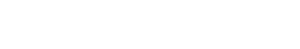Drag

# 机器学习代写｜MASC499 Spring 2021 Project #1

## Task #1 : Linear Regression

Objective: Perform linear regression test to model the true function, e.g. y = 2*x + 3, and observe
how noise in data affect the model training and prediction.

Record the number of epochs to converge, weight values (w0 & w1) by changing following
parameters. Summarize the results (use the table shown below) and write your observation in the
project report.

Noise level (noise_level) : 1, 5
Number of samples (num_data) : 20, 50, 100
Learning rate (alpha) : 1e-4, 1e-3, 1e-2
Convergence threshold (threshold) : 1e-9, 1e-6, 1e-3## Task #2 : Polynomial regression

Objective: Perform polynomial regression to model a sine curve data with noises. Observe how
noise in data affect the model training and prediction.

Record the mean square error (MSE) for training and test dataset as a function of the polynomial
order, the number of samples, and noise level. Summarize your results (like the table shown below)
and write your observation in the project report.Polynomial order : 1, 3, 5, 9
Number of samples (num_data): 20, 50, 100
Noise level: 0.1, 0.3, 0.6

Sample outputs: